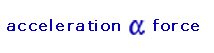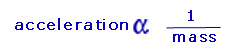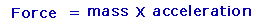## Newton’s Second Law of Motion

Newton’s first law deals with objects at rest or objects moving at constant velocity. His second law deals with the motion of accelerating and decelerating objects.

We know from everyday life examples such as pushing a car that if two people push a car on a flat road it will accelerate faster than if one person was pushing it. Thus, there is a relationship between the size of the force and the acceleration. We also know that it is easier for two people to push a small car than a large truck and for the same applied force the small car will accelerate faster than a large truck. Therefore there is also a relationship between mass and acceleration.

Experimentation proves that acceleration of a body is proportional to the force applied. This means that acceleration doubles when the force doubles or acceleration trebles when the force trebles, thus the greater the force the greater the acceleration. This relationship is represented as:(where α represents "directly proportional to")

Experiments also show us if you keep the force constant and double the mass, the acceleration will halve. This means the acceleration is inversely proportional to the mass i.e. the greater the mass the less the acceleration.It is important to note that the acceleration is parallel to the force applied.

Combing these two results we get:Rearranging the above equation:This equation gives the mathematical form of Newton’s second law of motion,
which states:

The acceleration of an object is inversely proportional to the mass of the object and directly proportion to the force acting on the object

Provided the force is measured in newtons the second law can be written mathematically as:The unit for measuring force is the newton. One newton is defined as:

The force which gives a mass of 1kg an acceleration of 1 m/s2

The animation below explains Newton’s second law of motion:

### Force Acting in Free Fall

The force acting on an object in free fall is called its weight. The acceleration of the object is due to the force of gravity, therefore from Newton’s second law of motion we know;

F = m x a

Acceleration due to gravity is represented by “g” and is equal to 9.8m/s2.

Therefore,

F = m x g
(where “g” is acceleration due to gravity)

We know that force acting due to gravity is weight so,

W = m x g